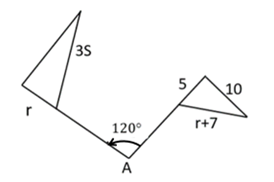Mathematics
Easy

Question

# What is the value of ‘r’ in the rotation of the triangle about A?## 3    4    5    6Hint:

## The correct answer is: 5

### Step 1 :   Rotation is isometry.r = 53s = r + 7 (substitute r = 5)Step 2 :   solving for s3s = 5 + 73s= 12s = 4.

corresponding sides are equal compare / equate them .

### Related Questions to study#### With Turito Foundation.#### Get an Expert Advice From Turito.